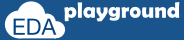# Blocking Assignment

Blocking assignment statements execute in series order. Blocking assignment blocks the execution of the next statement until the completion of the current assignment execution.

## Blocking assignment example

In Below Example, a and b is initialized with value 10 and 15 respectively, after that b is being assigned to a (a value will become 15), and value 20 is assigned to b. After assignment value of a = 15 and b = 20.

```module blocking_assignment;
//variables declaration
int a,b;
initial begin
\$display("-----------------------------------------------------------------");
//initializing a and b
a = 10;
b = 15;

//displaying initial value of a and b
\$display("\tBefore Assignment :: Value of a is %0d",a);
\$display("\tBefore Assignment :: Value of b is %0d",b);

a = b;
b = 20;

\$display("\tAfter  Assignment :: Value of a is %0d",a);
\$display("\tAfter  Assignment :: Value of b is %0d",b);
\$display("-----------------------------------------------------------------");
end```
```
endmodule```

Simulator output:
-----------------------------------------------------------------
Before Assignment :: Value of a is 10
Before Assignment :: Value of b is 15
After Assignment :: Value of a is 15
After Assignment :: Value of b is 20
-----------------------------------------------------------------

Execute the above code onExample-2:

In Below Example, a and b are initialized with value 10 and 15 respectively, after that b is being assigned to a (a value will become 15), and value 20 is assigned to b. After assignment value of a = 15 and b = 20.

```module blocking_assignment;
//variables declaration
int a,b;
int x,y;```
```  initial begin
//initializing a and b
a = 10;
b = 15;

x = a + b;
y = a + b + x;

\$display("-----------------------------------------------------------------");
\$display("\tValue of x is %0d",x);
\$display("\tValue of y is %0d",y);
\$display("-----------------------------------------------------------------");
end
endmodule ```

Simulator output:
-----------------------------------------------------------------
Value of x is 25  Value of y is 50
-----------------------------------------------------------------

Execute the above code on